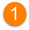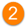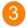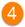• User Documentation
• API
• SDK
• Support

Search the Aria Knowledgebase for
User Documentation, APIs, SDKs, and more!

# Salesforce Field Calculations

## Overview

Listed below is information on how the MRR, ARR, TCV, and ACV fields in Salesforce are calculated.

## Salesforce Field Calculations

The corresponding fields are calculated as explained below.MRR Total – The cost of the plan per month.TCV – The total contracted value (activation + MRR for contracted months). The TCV is only calculated if a contract is applied. Otherwise, the TCV is \$0.ACV – The annual contracted value (activation + MRR for 12 months). The ACV is only calculated if a contract is applied. Otherwise, the ACV is \$0.ARR – The annual cost of the plan.

## Example of Calculations

Below is an example plan ("Plan A") and how the MRR, TCV, ACV, and ARR would be calculated.

"Plan A" Details:

Billing Interval: Quarterly

Recurring Cost: \$120 p/ quarter

Activation Service: \$100

Contract Applied: 6 month

Calculations:

MRR: 120/3 = \$40

ARR: \$40 x 12 = \$480

TCV: 100 + (40 x 6) = \$340

ACV: 100 + (40 x 12) = \$580

Note: For an annual contract, the TCV, ACV, and ARR will be equal if the plan does not have an activation service. Otherwise, the TCV and ACV will be equal.